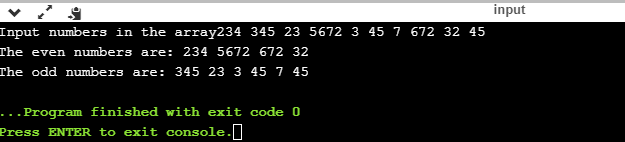# How to separate even and odd numbers in an array in C++

This tutorial will explain how to separate even and odd numbers in an array in C++ and transport them to two different and separate arrays. Illustration – Suppose we enter an array that contains the following elements 1,2,3,4,5,6,7,8,9 then the program will separate it into two extra arrays – Odd: 1,3,5,7,9 and Even: 2,4,6,8.( This should be kept specifically in mind that there needs to be the equal size of all the three arrays. Otherwise, a problem might arise later if all the numbers the user inserts are either even or odd and there’s not enough space in the array )

For those of you who don’t understand the concept of array its basically a type of contiguous data structure that has memory locations next to each other. This allows a rigid type of data structure whose length or position in memory cannot be altered once it is declared.

## separate even and odd numbers in an array in C++

```Input: 2324,2332,4,234,2,1,343,23,2345,1212,33,2

Output: Even array : 2324,2332,4,234,2,1212,2

Odd array  : 1,343,23,2345,33```

So this is fairly an easy program. Now we are going to see the algorithm of the program.

#### Algorithm of the program:

1. Take input in the form of an integer or float array(Arr).
2. Take evncnt=0 and oddcnt=0
3. start for loop i=0 up to i<= length of array
4. if (Arr[i]%2==0) then
5. even[evncnt++]=Arr[i]
6. else odd[oddcnt++]=Arr[i]
7. end if
8. end for
9. end

## Program to separate even and odd numbers in an array in C++

```#include <iostream>
using namespace std;
int main()
{
int arr,even,odd,evncnt=0,oddcnt=0,i;
cout<<"Input numbers in the array";
for(i=0;i<10;i++)
cin>>arr[i];
for(i=0;i<10;i++)
{
if(arr[i]%2==0)
even[evncnt++]=arr[i];
else
odd[oddcnt++]=arr[i];
}
cout<<"The even numbers are: ";
for(i=0;i<evncnt;i++)
cout<<even[i]<<" ";
cout<<"\nThe odd numbers are: ";
for(i=0;i<oddcnt;i++)
cout<<odd[i]<<" ";
}```

#### The output of the above program:As you can see the results came out pretty easily but there are few downsides of using this program using the array as we cannot determine how many numbers the array should hold in the first place since the array is not dynamic in c++. We also cannot change the size of the even and odd array thereby them taking equal space as of the primary array.

This is a very simple program. I tried my best to do it in the easiest way possible. Hope you like it. If you have any doubts, please comment below.